# Perpendicular Bisector: Definition, Theorem & Equation

An error occurred trying to load this video.

Try refreshing the page, or contact customer support.

Coming up next: Proof by Contradiction: Definition & Examples

### You're on a roll. Keep up the good work!

Replay
Your next lesson will play in 10 seconds
• 0:01 Definition
• 0:53 Creating a…
• 1:29 Math Applications
• 3:18 Real-World Examples
• 4:44 Lesson Summary

Want to watch this again later?

Timeline
Autoplay
Autoplay
Speed

#### Recommended Lessons and Courses for You

Lesson Transcript
Instructor: Elizabeth Often

Elizabeth has taught high school math for over 10 years, and has a master's in secondary math education.

How can we draw a triangle that will have two exactly equal length sides? Or what if we need to find the center of a circle that passes through a given set of points? In this lesson, we'll learn about the perpendicular bisector and how useful it can be in geometry!

## Definition of a Perpendicular Bisector

How can an archaeologist determine the size of a plate if only a piece of it has been found? How can a landscaper determine sprinkler placement for the most effective water use? It turns out that a single line, called the perpendicular bisector, can be very useful in both of these problems.

The perpendicular bisector of line segment AB is a line that does two things:

• Cuts the line segment AB into two equal pieces or bisects it
• Makes a right angle with the line segment AB (is perpendicular)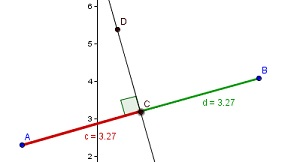An important property is that every point on the perpendicular bisector is the same distance from point A as it is from point B.

## Creating a Perpendicular Bisector

Although you can easily create a perpendicular bisector using geometry software, to do it on paper, all you need is a straightedge and a compass. First, draw your line segment, AB. Then use your compass to create a circle that has point A as its center, and passes through point B. After drawing this circle, draw a second circle that has point B at its center, and passes through point A. The two circles will intersect at two locations, as shown in the picture. A line drawn through the two points of intersection is the perpendicular bisector of the line segment.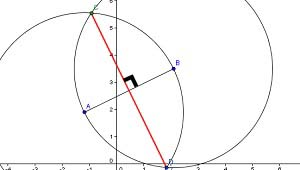## Math Applications

Most applications of the perpendicular bisector are in geometry theorems, proofs, and constructions. For example, you can use a perpendicular bisector to construct a triangle that has two equal length sides, known as an isosceles triangle. If you construct the perpendicular bisector of the line segment AB, every point on the perpendicular bisector will be the same distance from both point A and point B. To construct your isosceles triangle, you can start from any point on the perpendicular bisector and draw line segments to point A and to point B. The two line segments you have just drawn are guaranteed to be the same length! Additionally, the fact that a given line is a perpendicular bisector of one side of a triangle, and passes through the opposite vertex, is proof that the triangle is isosceles.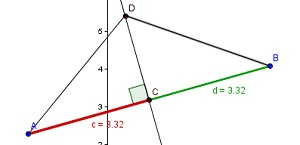You can also use the perpendicular bisector to find the circumcenter of a triangle. This point is the center of a circle that passes through all three corners, or vertices, of a triangle. To find this point, you will construct three perpendicular bisectors, one for each side of the triangle. The point where all three perpendicular bisectors intersect is called the circumcenter. Using this center point, we can draw a circle that passes through all three vertices.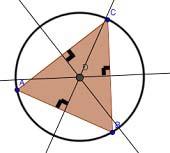Perpendicular bisectors are also useful in finding the center of a circle. If we are given three points on the circle, point A, point B and point C, then we can draw two line segments, AB and AC. The perpendicular bisectors of these two line segments will always intersect at the center of the circle.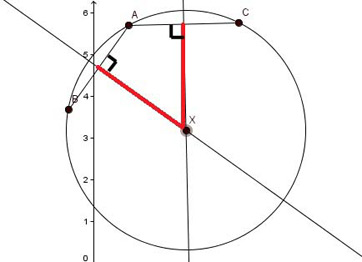## Real-World Examples

Installing a sprinkler system

To unlock this lesson you must be a Study.com Member.

### Register to view this lesson

Are you a student or a teacher?

### Unlock Your Education

#### See for yourself why 30 million people use Study.com

##### Become a Study.com member and start learning now.
Back
What teachers are saying about Study.com

### Earning College Credit

Did you know… We have over 160 college courses that prepare you to earn credit by exam that is accepted by over 1,500 colleges and universities. You can test out of the first two years of college and save thousands off your degree. Anyone can earn credit-by-exam regardless of age or education level.